# Catch up on the latest AI articles# Know The Best Trained Model With High Accuracy And Speed! Now Comes LEEP, A Metric For Predicting Model Metastability!

3 main points
✔️ Proposes LEEP, a metric that predicts with high accuracy which learned models should be used for transfer learning to produce accurate models
✔️ Faster computation because we only need to make predictions once for data in the target domain using the learned model
✔️ The first metric that shows a high correlation with the accuracy of recently proposed Meta-Transfer Learning

written by Cuong V. NguyenTal HassnerMatthias SeegerCedric Archambeau
(Submitted on 27 Feb 2020 (v1), last revised 14 Aug 2020 (this version, v2))
Comments: Accepted to the International Conference on Machine Learning (ICML) 2020.

Subjects: Machine Learning (cs.LG); Computer Vision and Pattern Recognition (cs.CV); Machine Learning (stat.ML)code：## First of all

As we all know, deep learning models have been used in various aspects of our daily life due to their very good prediction accuracy compared to conventional methods. In particular, in the fields of image recognition, natural language processing, and speech recognition, deep learning models have achieved overwhelming accuracy over conventional methods. One of the reasons why deep learning models have such good accuracy is that the models have acquired a mechanism to extract features that are very important for prediction.

On the other hand, it is known that the mechanism for extracting these features is acquired by training on a large amount of data. This means that a large amount of data is required to achieve accuracy in deep learning models. In other words, a large amount of data is required to achieve accuracy in deep learning models. Transfer learning " is a very effective method to solve this problem. Transfer learning makes it possible to achieve accuracy even with a limited amount of data by reusing the feature extraction part of a model that has been trained on a large amount of data. In general, the parameters of a model trained on 10 million images of 1000 classes called ImageNet (hereinafter referred to as "trained model") are reused, and only the part that actually performs prediction is replaced and relearned.

Now, this brings us to one question. What is the best-trained model for my data set? Here, we assume that the data to train the original learned model is fixed (such as ImageNet above). For example, ResNet, which solves the gradient vanishing problem of deep learning models, and MobileNet, which can run on CPU.

Of course, if you have enough computational resources, you can relearn using all possible models and choose the one with the best accuracy among them. However, general companies do not have such abundant computational resources, and they have no choice but to select and relearn models that they think are good within their limited computational resources. If it is possible to find a sub-optimal model with a small amount of computation, it would be very beneficial for companies. Given a trained model and a target dataset (a dataset to be re-trained), the goal of this research is to select a sub-optimal trained model that achieves good accuracy on the target dataset with a small amount of computation. We have proposed LEEP as a metric for this purpose, and have succeeded in selecting the optimal model with higher accuracy than conventional methods. Let's take a look at what LEEP is and how it works.

## Related research

### Metatransfer learning

Meta-transference learning is a very new research area proposed in 2018. The goal of meta-transference learning is to learn how to transfer from a source task to a target task. It may not sound quite right when expressed in letters, but research in educational psychology has shown that this is something that humans do on a daily basis. For example, let's consider a child who is good at chess. That child learns that the experience gained in chess can actually be applied to math and puzzles. As the child grows, he or she will have many experiences (building a desk with tools, playing nicely with friends, etc.) and when faced with a new and unknown task A, he or she will be able to use what he or she has learned to determine what task B can be used to help solve task A and how. In other words, we can learn how to transfer between tasks. In other words, they are learning how to transfer between tasks. The following figure shows the difference between transfer learning, multi-task learning, continuous learning and meta-transfer learning, and experiments have shown that LEEP is a good indicator of the accuracy of this meta-transfer learning.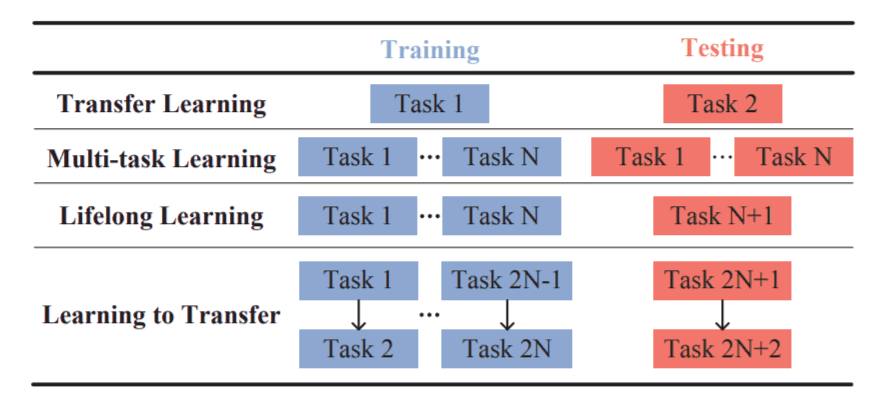Task space representation is an attempt to represent a very ambiguous thing called a task by a vector. For example, a method called TASK2VEC is famous and has been reported in AI-SCHOLAR. It represents tasks as vectors, and by measuring the distance between the vectors, it is possible to determine which dataset the model trained on should be used for transfer learning. However, TASK2VEC obtained a representation of the task by training a large model called a probe network and applying it to the target data. This means that it has the disadvantage of requiring ample computational resources.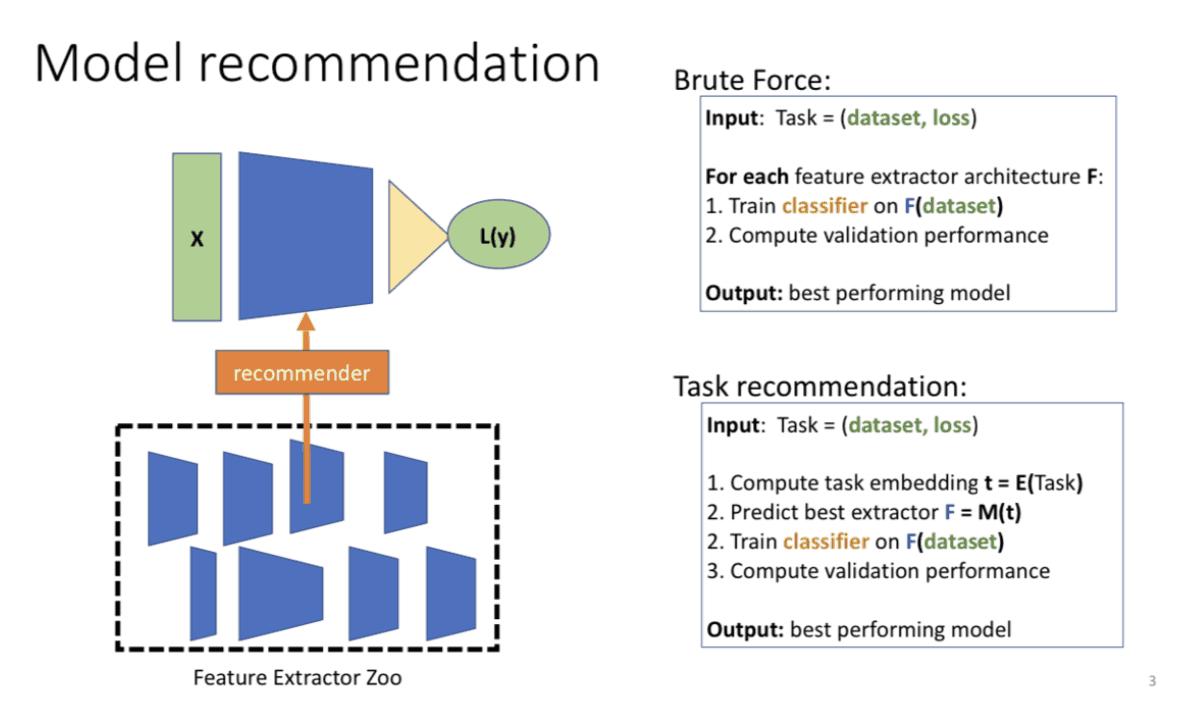## Proposed method

Now we will look at the proposal method. The proposed method consists of three steps.

(i) Compute the dummy label distribution of the target dataset with the learned model.
(ii) Calculate the conditional probability $P(y|z)$ for dummy label z.
3) Calculate LEEP from the dummy label distribution and $P(y|z)$.

We'll look at each of these steps in detail.

(1) Calculate the dummy label distribution of the target dataset with the learned model.

Let $θ$ be a trained model. For example, $θ$ is a model trained in Imagenet. The output of this model represents z. We also denote the target dataset as D={(x1,y1),(x2,y2)...(xn,yn)}. Here, we use $θ$ to compute the dummy label distribution $θ(xi)$ of the target dataset. Of course, $θ(xi)$ is semantically unrelated to the labels of the target dataset because we have not made any changes to the trained model.

(ii) Compute the conditional probability $P(y|z)$ for dummy label z

To calculate the conditional probability $P(y|z)$ for dummy label z, we first calculate the simultaneous probability distributions of y and z. The calculation formula is as follows.To calculate the simultaneous probability distribution of y and z, we are taking the sum of all dummy label distributions that satisfy yi=y. In other words, we are calculating the distribution of the data given the label y.

If we can calculate the simultaneous probability distribution, we can then follow Bayes' theorem to calculate We can calculate the conditional probability $P(y|z)$. From Bayes' theorem The conditional probability $P(y|z)$ can be calculated as follows.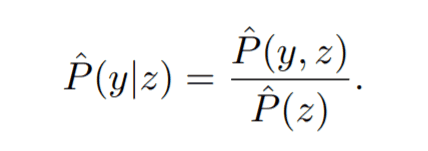where the denominator can be calculated from the simultaneous probability distribution as follows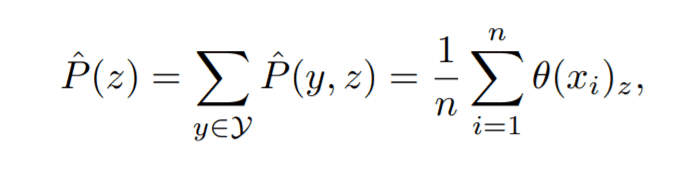(3) Dummy label distribution and calculate LEEP from $P(y|z)$.

We have calculated $θ(x)$ and $P(y|z)$, let's consider a classifier that predicts the label of x. First, we compute the label z from the dummy label distribution. Then, using the label z Compute y from $P(y|z)$. This means that $P(y|x,θ,D)$ = $ΣP(y|z)θ(x)$, which is equivalent to computing y from the probability distribution (Σ is computed for all z.) LEEP is defined by taking the log of this EEP and averaging it over the target data set.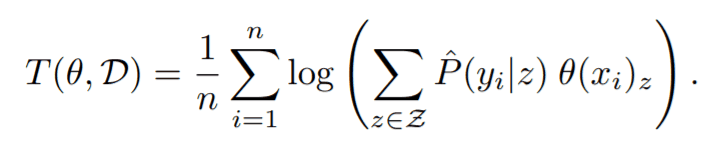Intuitively, LEEP is computed on a trained model $θ$ and a target dataset D and is a measure of how close $θ$ is to D. By looking at the theoretical properties of LEEP, we can see how right this part is.

### Theoretical consideration

First, we decompose $θ$ into a feature extraction mechanism $ω$ and a classifier $h$. In other words, $θ$=($ω$,$h$). Next, we consider replacing only the classifier part and retraining it in D. In this, we consider the problem of choosing the optimal classifier. This can be expressed by the following equation.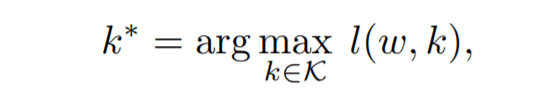where $l$ is the average log-likelihood in D and $K$ is the set of classifiers k. Assuming that $K$ contains the EEP, it follows that T($θ$, D)≤$l($ω$,k*)$- property (1). This implies that T($θ$,D)≤$l($ω$,k*)$-such that $l($ω$,k*)$ is the maximum of the mean log-likelihood and Since $T($θ$,D) is contained in$K$, property (1) holds. This means that LEEP is a lower bound on the maximum of the average log-likelihood. The following properties illustrate the relationship between LEEP and a recently published measure of metastability, negative conditional entropy (NCE), which has been shown to be a good measure of metastability. For every input xi in the target dataset D, we calculate the dummy label zi=argmax$θ(xi)$, where Y=(y1,y2...yn) and Z=(z1,z2...zn), as follows.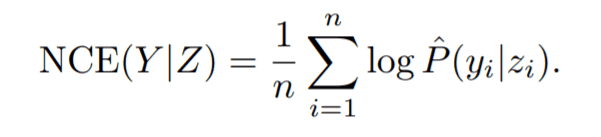Between this NCE(Y|Z) and LEEP, T($θ$,D)≥NCE(Y|Z)+$Σlogθ(xi)$/n - property (2) holds. (See Appendix of the original paper for illumination.) From properties (1) and (2), LEEP is the mean log-likelihood in the optimal model ($ω$,k*) in D andNCE(Y|Z)+$Σlogθ(xi)\$/n. Since the mean log-likelihood is known to be correlated with the accuracy of the model, and moreover has been proven to be closer to the mean log-likelihood than the NCE (as proved in the NCE study), we can prove that LEEP has a certain correlation with the accuracy of the model.

## Experiment

### LEEP vs. Transfer Accuracy

In this experiment, we use ResNet18 trained on ImageNet and ResNet20 trained on CIFAR10 as trained models. We transfer train these two models to CIFAR100. In this experiment, we compare the accuracy and LEEP of the transfer-trained models on 200 different tasks (we randomly select 2~100 classes from CIFAR100 and iterate them to build 200 different datasets). In this section. We are experimenting with two types of models: Re-train head (replacing only the classifier and retraining only the classifier part) and Fine-tune (replacing the classifier and retraining the whole model). See the figure below. We can see that for both trained models, the accuracy of LEEP and the re-trained model are highly correlated.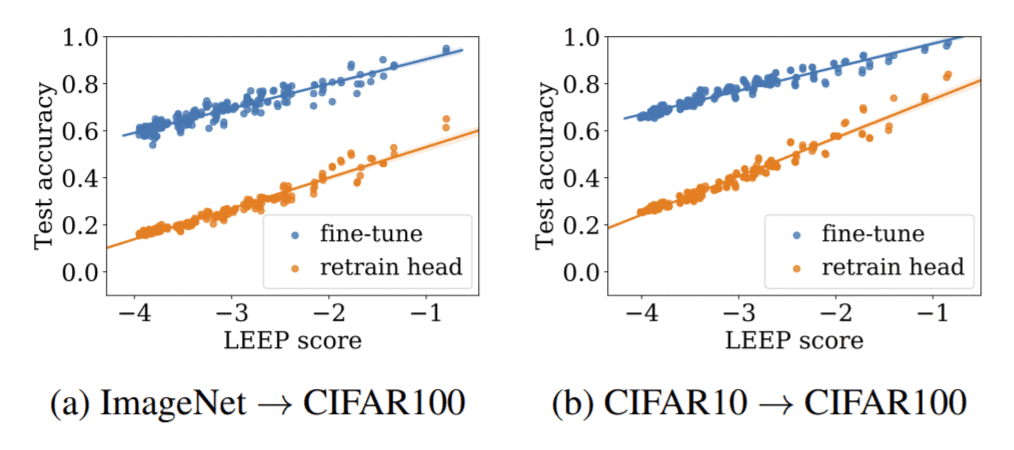### LEEP vs. Convergence of Fine-tuned Models

In the next experiment, we use LEEP to predict the speed of convergence of fine-tuned models. to measure the speed of convergence of fine-tuned models, we randomly select five classes from the dataset as Reference models and train them from scratch using all the samples in the classes. The fine-tuned models are selected from the same five classes as above from CIFAR100, and each class is trained with 50 images. Please see the following figure. The black dotted line (0.0) in the figure is the area where the difference in accuracy between the scratch model and the Fine-tune model is 0. In addition, level1~5 means that the value of LEEP is divided into five levels, and the larger the level, the larger the value of LEEP. The following figure shows that the convergence becomes faster as the LEEP value becomes larger.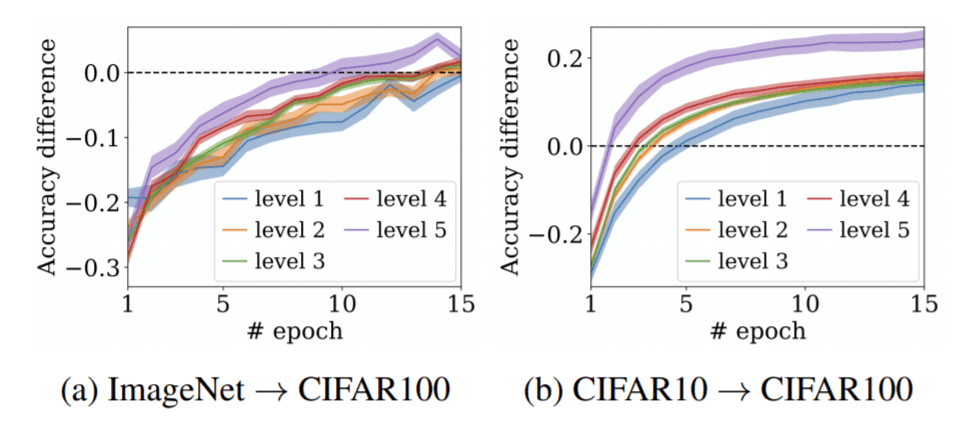### Comparison of LEEP, NCE, H scores, CNAPS

In the next set of experiments, we investigate the correlation between the values of the latest metrics of transfer learning (NCE, H scores) and the latest methods of meta-transfer learning, CNAPS and LEEP. In this experiment, we add restrictions to the target dataset D: large indicates retraining using the entire dataset, small indicates retraining using only a part of the dataset, and imblanced indicates varying the number of samples in each class from 30~60. From the following figures, we can see that LEEP shows a high correlation compared to the latest methods.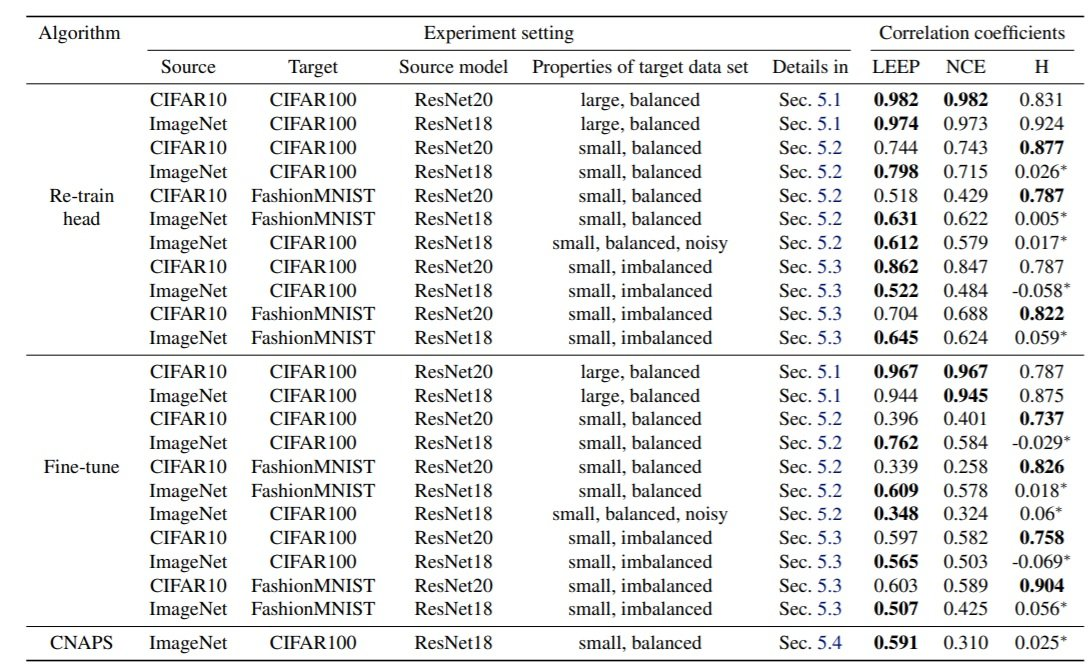### LEEP for Source Model Selection

This is where the subject of this paper comes in: model selection using LEEP. The trained models we used are ResNet, MobileNet, SENet, and DarkNet. As you can see in the figure below, LEEP accurately predicts the accuracy of the models compared to the other methods. In other words, the accuracy of the model is highly correlated with the value of LEEP, so if a model with a high LEEP value is used, the accuracy of the retrained model is predicted to be high. (For example, NCE does not predict MobileNet1.0 well.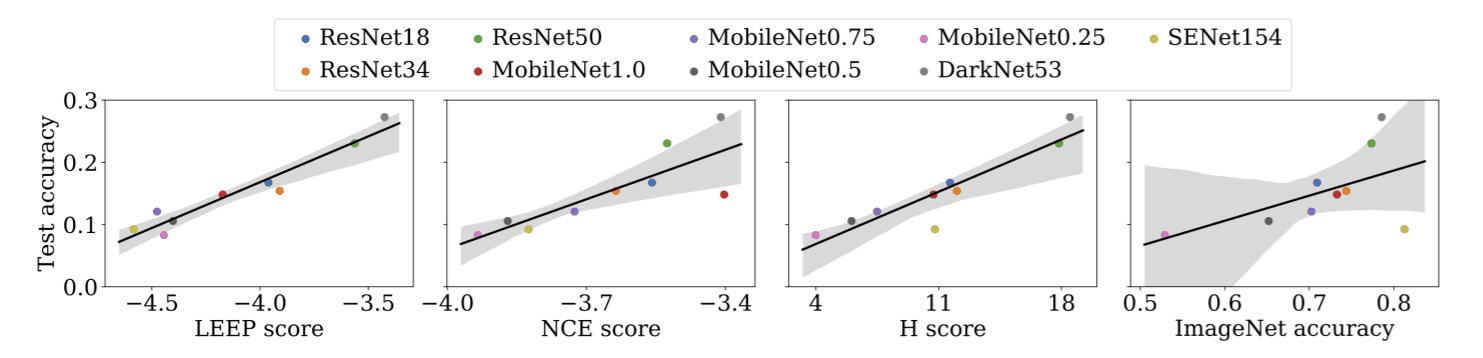## Summary

The work presented in this paper proposes a metric, LEEP, that predicts the optimal learned model efficiently and with high accuracy. The experimental results show that the LEEP values for nine different models are highly correlated with the accuracy of re-training on each model. In other words, by using LEEP, we can select a suboptimal model architecture with limited computational resources. Thus, transfer learning is a very important technique in the use of deep learning models. In this transfer learning, such a technique that can predict which learned model to choose without training would be very useful. This is a very interesting area of research, so why don't you check it out?

If you have any suggestions for improvement of the content of the article,